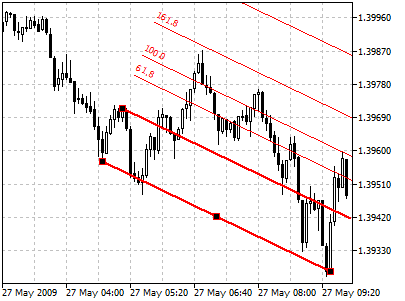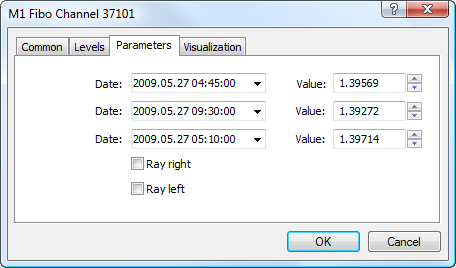# 菲波纳奇通道

## 绘制## 参数

For Fibonacci Channel construction trendline construction settings can be changed. 此外，该物件有以下参量：• Date/Value ― coordinates of the first point on the main line of Fibonacci Channel (date/value of the price scale);
• Date/Value ― coordinates of the last point on the main line of Fibonacci Channel (date/value of the price scale);
• Date/Value ― coordinates of the point on the second line of Fibonacci Channel (date/value of the price scale);
• Ray Right ― infinite duration of Fibonacci Channel to the right;
• Ray Left ― infinite duration of Fibonacci Channel to the left.